# Arithmetic with Complex Numbers

We’ve seen what complex numbers are; now we can look at what we can do with them. The basic operations are not hard, but have a few interesting features related to graphs. So that’s where we’ll start

## Graphing complex numbers

Graphing will provide a basis for some of the ideas we see here, but also tie it back to last week’s explanation of what complex numbers really are, mathematically (i.e. ordered pairs). Here is a question from 1997:

Graphing Complex and Imaginary Numbers

How do you graph imaginary numbers?

Here's how it goes:

Since any complex number z = a + ib has two real numbers associated with it, we can associate with each complex number z = a + ib an ordered pair of real numbers (a,b). Why is it an ordered pair? Because the order is important: the ordered pair (2,3), for instance, corresponds to the complex number 2 + 3i, and the ordered pair (3,2) corresponds to the complex number 3 + 2i, which is different from 2 + 3i.

Does that remind you of something?  I hope it reminds you of the regular old xy plane, where you have two coordinate axes (the x and y axes), oriented perpendicular to one another, and a point in the plane is associated with an ordered pair of numbers (a,b), where the number a gives the x coordinate of the point, and the number b gives the y coordinate of the point.

I want to add here that, in $$a+bi$$, the real number a is called the “real part” of the number (notation: $$\text{Re}(a+bi)=a$$), and the real number b is called the “imaginary part” of the number (notation: $$\text{Im}(a+bi)=b$$). I used to think the imaginary part ought to be … imaginary (i.e. bi)! But these are the real numbers that form the coordinates, or components, of the ordered pair.

Here are the numbers $$2+3i$$ and $$3+2i$$: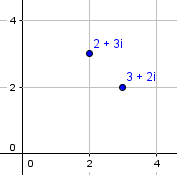Such a graph is sometimes called an Argand diagram.

Now notice that if we have a complex number z = a + ib, we can associate with it the ordered pair of numbers (a,b), and that is associated with the point whose x coordinate is a, and whose y coordinate is b. This allows us to associate with every complex number a point in the xy plane. Similarly, every point in the xy plane is associated with an ordered pair of numbers (a,b), and we can associate with this ordered pair the complex number z = a + ib.

Thus, every complex number is associated with a point in the xy plane, and every point in the xy plane is associated with a complex number.  This allows us to represent every complex number as a point in the xy plane, and vice-versa.  When we do this, we call the xy plane the *complex plane*.

So we’re thinking of the complex numbers as being points on this plane. And that’s just what the construction we saw last week did.

For instance, the complex number 2 - 3i (= 2 + (-3)i) can be represented as the point (2,-3). The complex number 4i = 0 + 4i can be represented as the point (0,4). The *real* number 2 = 2 + 0i (since it is still true that 0 times any number (real *or* complex) is 0, the number 0i is just equal to 0) can be represented by the point (2,0).  Do you see that real numbers (numbers of the form a + 0i) correspond to points on the "x" axis, and that purely imaginary numbers (numbers of the form 0 + bi, where b is real) correspond to points on the "y" axis?

Here are $$2-3i$$, $$4i$$, and $$2$$: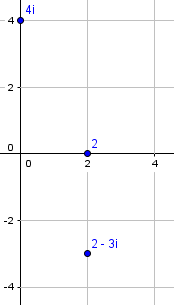As a result, when we talk about the complex plane, we rename the x and y axes: the x axis is now called the "real axis" (since that is where all the points correspond to real numbers), and the y axis is now called the "imaginary axis" (since that is where all the points correspond to purely imaginary numbers).

We’ll continue with this 2002 question:

A Primer on Complex Arithmetic

I really don't understand how to use complex numbers. How do I do ones like this: (4-10i)(4+10i)?

Ones like this are even worse:

w = 3-4i
z = 3+4i

Please help me!

The specific questions have a special feature (product of conjugates), which actually makes them simpler; but Doctor Ian chose to answer the bigger question of how all four arithmetic operations are done, not just these particular multiplications.

Hi Lindsey,

Here's a quick primer on complex arithmetic.

For purposes of adding and multiplying, you can think of complex numbers as binomials, like (x - 2) or (x + 5).

To add (2x - 2) and (3x + 5), you just add the coefficients of like terms:

(2x - 2) + (3x + 5) = x(2 + 3) + (-2 + 5)

= 5x + 3

(Similarly for subtraction.)

So to add complex numbers, too, we add like terms (real and imaginary components): $$(-2+2i)+(5+3i)=-2+5+2i+3i=(-2+5)+(2+3)i=3+5i$$

As for subtraction: $$(-2+2i)-(5+3i)=-2-5+2i-3i=(-2-5)+(2-3)i=-7-i$$

Just treat i as if it were a variable, distributing and combining terms as usual.

### Multiplying

To multiply (x - 2) and (x + 5), you just apply the distributive property a couple of times:

(x - 2)(x + 5)

= x(x + 5) - 2(x + 5)

= x^2 + 5x - 2x - 10

= x^2 + 3x - 10

Same thing with complex numbers:

(2 + 3i)(4 - 5i)

= 2(4 - 5i) + 3i(4 - 5i)

= 8 - 10i + 12i - 15i^2

= 8 - 2i - 15i^2

What makes this nicer, though, is that the i^2 term disappears, because i^2 is just equal to -1,

= 8 - 2i + 15

= 23 - 2i

giving you a new complex number.

So, again, we just treat i as a variable, with the one additional fact that its square can be replaced with $$-1$$, so in the final answer, no powers of i will ever be needed. Multiplication just means multiplying each term in the first by each term in the second, often called “FOIL”.

### Conjugates

Now we come to the special case represented by the particular question that was asked, the product of conjugates (which we’ll see again later):

Do you remember this pattern?

(x + a)(x - a) = x^2 - a^2 ?

It works for complex conjugates, too:

(3 + 4i)(3 - 4i) = 3^2 - (4i)^2

= 9 - (4^2 i^2)

= 9 - (-16)

= 25

So when you multiply a complex number by its conjugate, you get a real number.

As we’ll see, this property will be very useful. In general, $$(a+bi)(a-bi)=a^2+b^2$$

### Dividing, the hard way

He takes an unusual approach to division, one that you might try for yourself if you had no idea what to do, but is not the final method we’ll see later:

Division looks scarier, but it's kind of like factoring. Suppose you want to find

26 - 7i
-------
3 + 4i

When you want to factor something like x^2 - 2x -6, you start by assuming that it will look like (x + a)(x + b), and then you figure out what a and b are, right? Same thing here. You assume that you're going to end up with (a + bi), and then you use the definition of division:

26 - 7i
------- = a + bi
3 + 4i

26 - 7i = (a + bi)(3 + 4i)

= 3a + 4ai + 3bi + 4bi^2

= (3a - 4b) + (4a + 3b)i

^           ^
|           |
So this     So this
has to      has to
equal 26    equal -7

Does this look familiar yet?

Here he has simplified the equation by multiplying, to obtain a new equation. But for two numbers to be equal, the real parts and the imaginary parts of each must each be equal:

That gives you two equations in two unknowns,

3a - 4b = 26

4a + 3b = -7

which you can solve by elimination or substitution. I'll use elimination:

9a - 12b =  78           (Multiply the 1st eq. by 3)

16a + 12b = -28           (Multiply the 2nd eq. by 4)
-----------------
25a       = 50

So a = 2, and

3(2) - 4b = 26

6 - 26 = 4b

-20 = 4b

So b = -5, which means that

26 - 7i
------- = 2 - 5i
3 + 4i

This isn't the prettiest way in the world to do arithmetic. On the other hand, I'd rather do this than long division any day.

We’ll see a quicker method for division soon.

The most important thing is not to panic. Keep in mind that if you add, subtract, multiply, or divide two complex numbers, you're going to end up with another complex number; and remember that i^2 = -1, so the exponents don't keep growing the way they do with polynomials.

Now let’s look closer at addition, with this question from 2006:

Graphing Sums of Complex Numbers

Why is it that when two complex numbers are graphed, then the sum of those two complex numbers is graphed (all of this on the same graph), and then lines are drawn to connect the parts of each graph farthest from the origin, a parallelogram is formed?

I have a hunch that there is a proof for this, but if there is any other explanation that isn't a proof, I would be glad to have both that and the proof.

Michael has seen a graphical approach to addition, but missed the explanation! I answered:

Hi, Michael.

Addition of complex numbers is the same as addition of vectors, which follow this parallelogram rule as well.  In both cases, it works because addition is done by adding coordinates.

The direct way to think of addition is to represent each number by an arrow, and to put these arrows together head to tail, one after another.  To add (a+bi) + (c+di), you would do this:

|               o
|           /   |d
|       /       |
|    o----------+
|   /|     c    |
|  / |          |
| /  |b         |b
|/   |          |
+----+----------+--
a       c

Clearly the result is (a+c) + (b+d)i; we have added the x-coordinates and added the y-coordinates.

That is, when we add complex numbers, we just add the real parts, and add the imaginary parts.

Adding into the picture arrows parallel to these (essentially adding the same numbers in the opposite order, ending up at the same point), we get

|               o
|           /  /
|       /     /
|    o       /
|   /       /
|  /       o
| /     /
|/  /
+------------------

There's your parallelogram!

The top parts represent $$(a+bi)+(c+di)$$, and the bottom parts represent $$(c+di)+(a+bi)$$. Here is an example, $$(1+2i)+(3+i)=4+3i$$: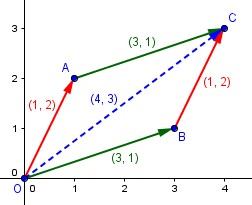To do the addition, we can plot the two numbers (A and B), then complete the parallelogram to find the sum (C).

Subtraction means adding the opposite (negative); here is $$(1+2i)-(3+i)=(1+2i)+(-3-i)=-2+i$$: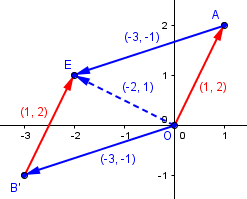We can also think of it as the other diagonal of the same parallelogram: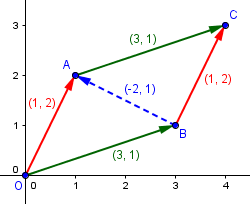## Complex conjugates

Before we dig deeper into division, here is a question from 2011:

The "What?" -- and "So What?" -- of the Complex Conjugate

My book lists some "basic concepts for complex numbers." One of the concepts states:

"x + yi and x - yi are mutual complex conjugates."

"8 + 3i and 8 - 3i are mutual complex conjugates."

I see that the only difference between the two expressions is the positive and negative signs, but I still don't know what a conjugate IS. Therefore, I don't know how to actually use or apply this information.

I do understand what a complex number is. And this book shows examples of mutual additive inverses, which I also understand.

I am doing this studying on my own to prepare for a math test, so I have no teacher to ask; and the "math people" I personally know do not know what it means, either.

Hi, Terri.

I thought at first that your issue was with the word "mutual," since that is the part of this term that I don't see much. However, since you understand the concept of mutual additive inverses, I suppose you do understand that this statement ...

"x + yi and x - yi are mutual complex conjugates"

... means that x - yi is the complex conjugate of x + yi, and that x + yi is the complex conjugate of x - yi. (The word "mutual" refers to something that is true of each of two or more parties in respect to the other.)

For example, each of $$2+3i$$ and $$2-3i$$ is the conjugate of the other, just as $$3$$ and $$-3$$ are mutual additive inverses, each the opposite (negative) of the other.

Two common notations for conjugates are $$\bar{z}=\overline{x+yi}=x-yi$$ and $$z^*=(x+yi)^*=x-yi$$.

The way to find the complex conjugate of a complex number is to negate the imaginary part of the number. That's what the statement says: if you've got a number of the form x + yi, then the complex conjugate of that number is x - yi. (Left unstated: x and y are real numbers.) For instance, for the number 8 + 3i, x is 8 and y is 3, so the complex conjugate is 8 - 3i. I think you're saying that you get this, too.

Perhaps what you're missing is the "so what?" I'll show you one important fact about complex conjugates that makes them useful enough to have a name.

Take the example: 8 + 3i and 8 - 3i. What is their product?

(8 + 3i)(8 - 3i) = 8*8 + 8(-3i) + 8(3i) + (3i)(-3i)
= 64 - 24i + 24i + 9
= 73

The product of these two complex numbers is a real number!

The same is true of any pair of mutual complex conjugates:

(x + yi)(x - yi) = x^2 + y^2

This is very similar to the conjugate of a radical expression, where we change $$a+\sqrt{b}$$ to $$a-\sqrt{b}$$. Just as the product of such an expression and its conjugate eliminates the radical, $$(a+\sqrt{b})(a-\sqrt{b})=a^2-b,$$ the product of a complex number and its conjugate eliminates the imaginary part. Both are used in similar ways:

One use of this fact is in dividing one complex number by another. For instance,

2 + 5i   (2 + 5i)(8 - 3i)   31 + 34i
------ = ---------------- = -------- = (31/73) + (34/73)i
8 + 3i   (8 + 3i)(8 - 3i)      73

I multiplied the numerator and denominator by the complex conjugate of the denominator; this made the denominator real, so I could distribute it across the sum, writing the result as the sum of a real part and a pure-imaginary part.

Does that help?

This is the standard way to do such a division.

Terri replied,

Yes, that helped so much!

And you had it exactly right when you suggested that perhaps my underlying question was "what's the point?" So not only did you help me with this particular question, I now know how to ask this type of question with other topics, as well! (In fact, I have another one I'm sending in about absolute value....)

Thank you so much for taking time out of your day to do this. I greatly appreciate it!

Incidentally, conjugates also do something interesting when you add them: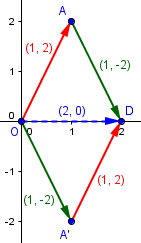This shows that the sum of any number (here $$1+2i$$) and its conjugate (here $$1-2i$$) is a real number, because the imaginary parts cancel one another.

Next week we’ll see the geometric meaning of multiplication, and get another perspective on why the product of conjugates is also real. For now, it’s just an algebraic fact.

## Dividing, the quick way

Here is a 2003 question:

Dividing by Complex Numbers

I'm having difficulty with problems that have an imaginary number but don't cancel. Example: Divide each pair of complex numbers: (8+4i)/(1+2i)

Any help would be great.

I suspect the part about canceling may mean that Ryan has seen a special case like this: $$\frac{8+4i}{2+i}=\frac{4(2+i)}{2+i}=4\cdot\frac{2+i}{2+i}=4$$ Such canceling doesn’t usually happen!

Doctor Luis answered, first explaining what we just saw about conjugates, and then continuing with a different example:

Knowing this fact about complex numbers, to divide you simply multiply and divide by the conjugate of the denominator.

Here's how:

20 + 30i     20 + 30i     -1 - 2i
--------- =  ---------- * ----------    (conjugate trick)
-1 + 2i       -1 + 2i     -1 - 2i

(20 + 30i)(-1 - 2i)
=  -------------------        (multiplying bottom)
(-1)^2 + (2)^2

= (40 - 70i)/5                (after multiplying top)

= 8 - 14i                     (final answer)

That's all there is to it. You make the denominator into a number you can divide by (that is, a real number), using complex conjugates. With this background, you should be able to solve the division you asked about.

Ryan’s own example is a little uglier, but more typical: $$\frac{8+4i}{1+2i}=\frac{8+4i}{1+2i}\frac{1-2i}{1-2i}=\frac{24-12i}{5}=\frac{24}{5}-\frac{12}{5}i$$

## Absolute value

We’ll close with this question from 1998:

The Absolute Value of a Complex Number

In our Alg II class, we were working with complex numbers (a + bi format). Our textbook and graphing calculators said that the absolute value of a + bi was the same thing as sqrt(a^2 + b^2). We couldn't understand where the i went. Could you help us?

Hi Russell -

One way of understanding absolute value is the following: the absolute value of a number is the distance from that number to zero. That is, if we think of a number as a point in the x-y plane, the absolute value of that number is the distance between that point and the origin.

Now, if the number is real, as a point it lies on the x-axis, so the distance from the origin to the number is just the usual absolute value.


This is the absolute value as we are used to thinking of it, on the number line: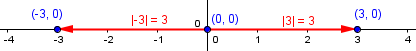But, if we take a complex number a + bi, it sits at the point (a,b) in the x-y plane. How far is (a,b) from (0,0)? Well, we can make a triangle to help us out:

draw a line (the hypotenuse) between the origin and (a,b);
draw a line from (a,0) to (a,b);
draw a line (a,0) to (0,0)

That gives us a right triangle that lies on the x-axis.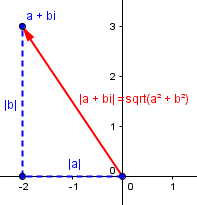$$|a+bi|=\sqrt{a^2+b^2}$$

Now, since it's a right triangle, we know that the length of the hypotenuse is the square root of the sum of the squares of the other sides.

How long are these other sides?

Well, one is the absolute value of a, and the other is the absolute value of b. (If a and b are positive, you don't need the absolute value, but we need to allow a and b to be negative, so that a + bi can be any complex number.)

Now, the Pythagorean theorem says that the distance from (a,b) to (0,0) is the square root of |a|^2 + |b|^2. But, |a|^2 = a^2 for all real numbers a. (Why? If a >= 0, then |a| = a, so it's true. If a < 0, then |a| = -a, so |a|^2 = (-a)^2 = ((-1)^2)*a^2 = (1)a^2 = a^2.)

So the distance from (a,b) to (0,0) is the square root of a^2 + b^2, and that's why it's the absolute value of a + bi.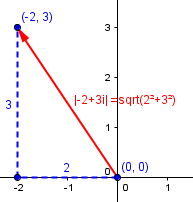$$|-2+3i|=\sqrt{(-2)^2+(3)^2}=\sqrt{13}$$

Notice that this formula works for real numbers as well as complex ones. If x is real, then x = x + 0i, and the absolute value of x is the square root of x^2 + 0^2, which is just the square root of x^2. What's the square root of x^2? The usual absolute value of x.

One more thing you might notice: We’ve seen the same expression before. In fact, $$(a+bi)(a-bi)=a^2+b^2=|a+bi|^2\\z\bar{z}=|z|^2$$

Next week we’ll see that a complex number can be described by its absolute value together with its direction, giving multiplication a new and important meaning.

This site uses Akismet to reduce spam. Learn how your comment data is processed.